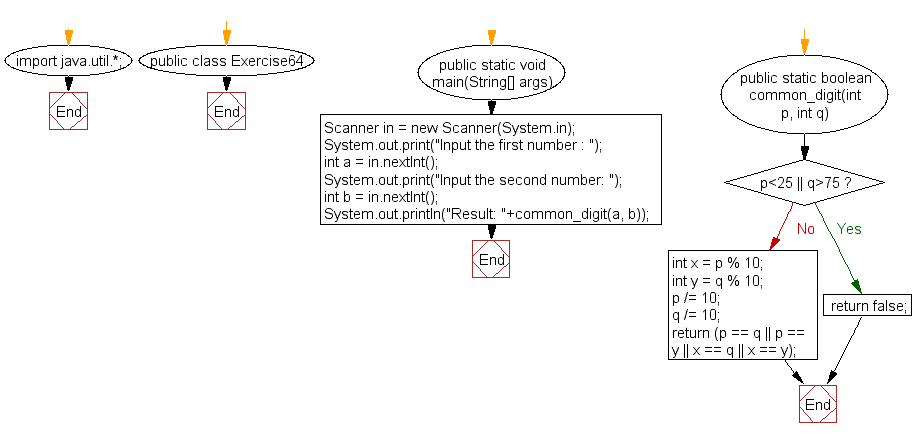﻿ Java: Test if 2 numbers have the same digit between 2 values# Java Exercises: Accepts two integer values between 25 to 75 and return true if there is a common digit in both numbers

## Java Basic: Exercise-64 with Solution

Write a Java program that accepts two integer values between 25 to 75 and return true if there is a common digit in both numbers.

Sample Solution:

Java Code:

``````import java.util.*;
public class Exercise64 {
public static void main(String[] args)
{
Scanner in = new Scanner(System.in);
System.out.print("Input the first number : ");
int a = in.nextInt();
System.out.print("Input the second number: ");
int b = in.nextInt();
System.out.println("Result: "+common_digit(a, b));
}

public static boolean common_digit(int p, int q)
{
if (p<25 || q>75)
return false;
int x = p % 10;
int y = q % 10;
p /= 10;
q /= 10;
return (p == q || p == y || x == q || x == y);
}
}
```
```

Sample Output:

```Input the first number : 35
Input the second number: 45
Result: true
```

Pictorial Presentation:Flowchart:Java Code Editor:

What is the difficulty level of this exercise?

Test your Programming skills with w3resource's quiz.

﻿

## Java: Tips of the Day

countOccurrences

Counts the occurrences of a value in an array.

Use Arrays.stream().filter().count() to count total number of values that equals the specified value.

```public static long countOccurrences(int[] numbers, int value) {
return Arrays.stream(numbers)
.filter(number -> number == value)
.count();
}
```

Ref: https://bit.ly/3kCAgLb